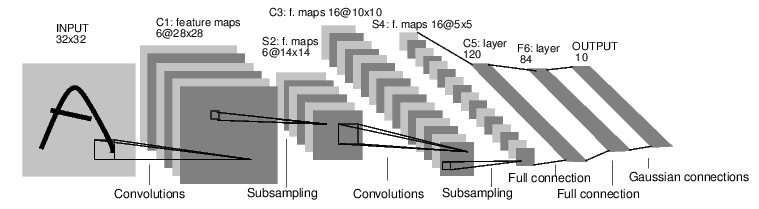PyTorch 是一个基于 Python 的科学计算包，主要定位两类人群：

``````NumPy 的替代品，可以利用 GPU 的性能进行计算。

``````

## 一、张量Tensors

Tensors 类似于 NumPy 的 ndarrays ，同时 Tensors 可以使用 GPU 进行计算。

## 二、自动微分

### 2.1 Tensor

``````tensor([[1., 1.],
tensor([[3., 3.],
``````

``````tensor([[27., 27.],
``````

``````False
True
<SumBackward0 object at 0x7fe1db427dd8>
``````

### 2.2 梯度

``````tensor([[4.5000, 4.5000],
[4.5000, 4.5000]])
``````

## 三、神经网络``````1.定义一个包含可训练参数的神经网络

2.迭代整个输入

3.通过神经网络处理输入

4.计算损失(loss)

5.反向传播梯度到神经网络的参数

6.更新网络的参数，典型的用一个简单的更新方法：weight = weight - learning_rate *gradient
``````